# scipy.spatial.distance.yule¶

scipy.spatial.distance.yule(u, v)

Computes the Yule dissimilarity between two boolean n-vectors u and v, which is defined as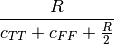where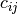is the number of occurrences of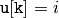and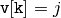for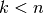and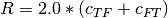.

Parameters : u : ndarray An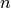-dimensional vector. v : ndarray An-dimensional vector. d : double The Yule dissimilarity between vectors u and v.

#### Previous topic

scipy.spatial.distance.sqeuclidean

#### Next topic

scipy.spatial.KDTree